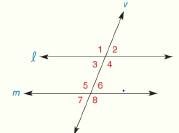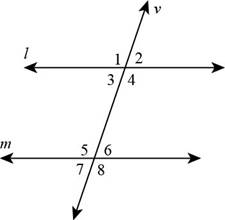Chapter 2.CT, Problem 1CTElementary Geometry For College St...

7th Edition
Alexander + 2 others
ISBN: 9781337614085

Solutions

Chapter
SectionElementary Geometry For College St...

7th Edition
Alexander + 2 others
ISBN: 9781337614085
Textbook Problem

Consider the figure shown at the right.a) Name the angle that corresponds to ∠ 1 .b) Name the alternate interior for ∠ 6 .To determine

a)

To find:

The angle corresponds to 1 in the given figure.

Solution:

The 5 is corresponds to 1.

Explanation

Given:

The following figure shows the given diagram.Calculation:

The angles that lie in the same relative positions are called corresponding angles for the given lines. Therefore, 5 and 1 lie in the same relative positions about the transversal. Therefore, 5 corresponds to 1.

Conclusion:

Hence, 5 corresponds to 1.

To determine

b)

To find:

The alternate interior angle for 6.

Solution:

The alternate interior angle for 6 is 3.

Explanation

Given:

The following figure shows the given diagram.Calculation:

Alternate interior angles are that angles which have different vertices and lie on the opposite side of the traverse. 6 and 3 have different vertices and lie on the opposite side of the transverse. Therefore, the alternate interior angle for 6 is 3.

Conclusion:

Hence, the alternate interior angle for 6 is 3.

The Solution to Your Study Problems

Bartleby provides explanations to thousands of textbook problems written by our experts, many with advanced degrees!

Get Started

Evaluate the expression sin Exercises 116. (23)2

Finite Mathematics and Applied Calculus (MindTap Course List)

If g(x)=f(x), where the graph off is shown, evaluate g(3).

Single Variable Calculus: Early Transcendentals, Volume I

In Exercises 35-38, find an equation of the line that has slope m and y-intercept b. 35. m = 3; b = 4

Applied Calculus for the Managerial, Life, and Social Sciences: A Brief Approach

True or False:

Study Guide for Stewart's Single Variable Calculus: Early Transcendentals, 8th

True or False: is a geometric series.

Study Guide for Stewart's Multivariable Calculus, 8th

Find all the answers to your study problems with bartleby.
Textbook solutions plus Q&A. Get As ASAP arrow_forward